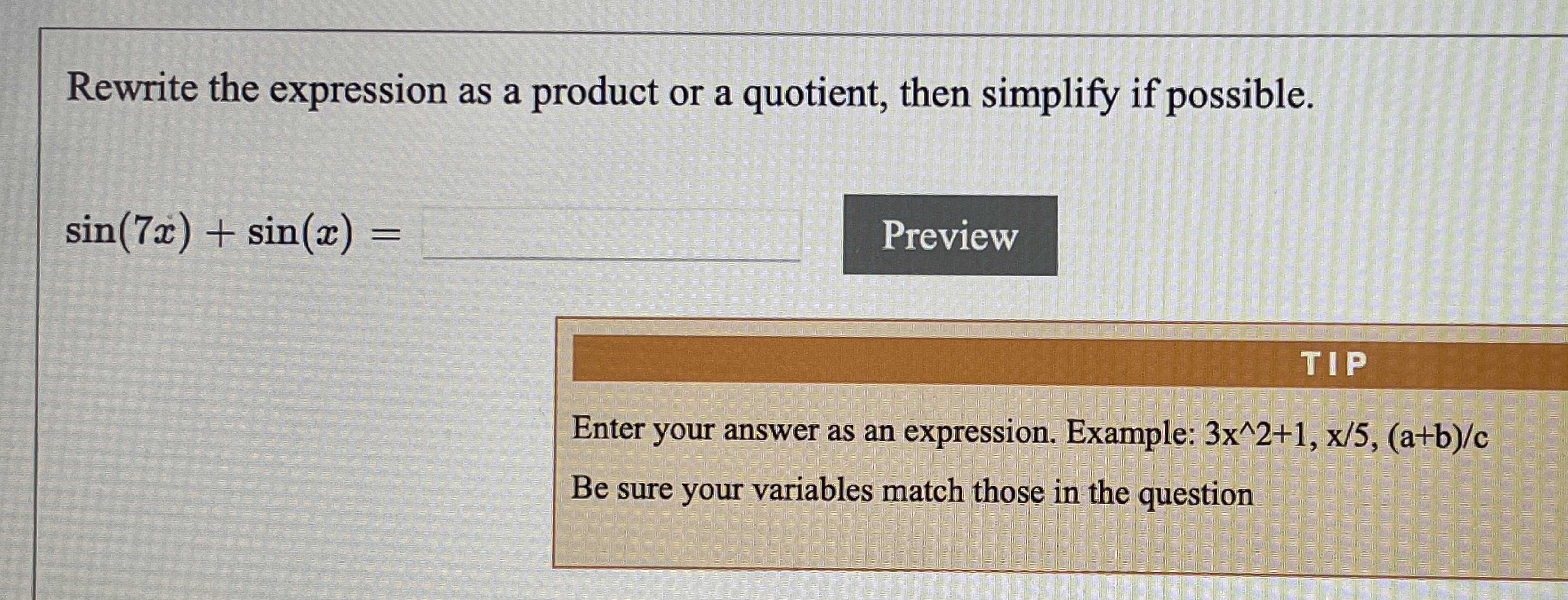### Still have math questions?

Trigonometry
QuestionRewrite the expression as a product or a quotient, then simplify if possible.

$$\sin ( 7 x ) + \sin ( x ) =$$ _______

Example: $$3 x ^ { \wedge } 2 + 1 , x / 5 , ( a + b ) / c$$
$$\sin ( 7 x ) + \sin ( x ) = 2\sin\frac{7x+ x}{2}\cos\frac{7x- x}{2}= 2\sin{4x}\cos{3x}$$